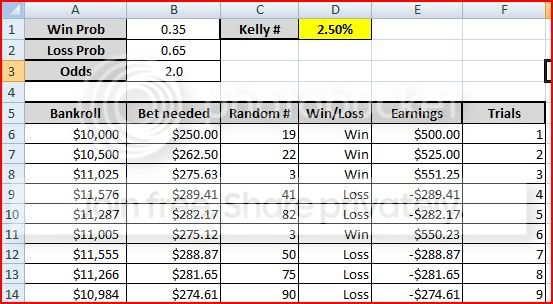# What if analysis of an Excel spreadsheet

Here is my set up.

I am running a simulation of a gambler betting over time

Here are the givens I am using.

Probability of win = 35% which is in B1 table

Odds = 2 which is in B3 table

Kelly # (amount of current bankroll to bet each time) = 2.5% which is in D1 table

Bankroll = $10,000 which is in A6 table I am going to put in formulas I have for other cells in my simulation of bets, but will provide the data that those tables hold as well SOME OTHER COLUMNS B6: =$D$1*A6 C6: =randbetween(1,000) column D6: =if(C6<36,"Win","Loss") E6: =if(D6="Win",B6*$B$3,(-B6)) A7: =A6+E6 Then I drag these down to run 2000 simulation of random bets Near the bottom I have a table that has the capital gain the final bankroll - the beginning$10,000 bankroll.

(is there a way to graph these wins on a line graph just the start of $10,000 to my final bankroll?) also what i need most How to make a what if analysis... comparing different outcomes if the Kelly # (the percentage of my current bankroll I am betting) was different. 0.01 0.015 0.02 0.025 0.03 0.035 0.04 and under each column of the different percentages I want the final capital gain in them... and I want to have like 200 examples of this new capital gain... Then I will average each column above for the 200 capital gains of the different percentages. My confusion is this... First off what is the value on the left of the percentages that I need to put in that block that will be concrete and unchanging? Is it$10,000 my beginning bankroll?

Then what are the values under that number?

I know I need to highlight the grid beginning with that concrete block (set as $10,000?) and then highlight down the 200 simulations I want. Then I go to data > what if analysis > data table Now what do i put in the table for row input cell? what about for the column input cell? <--- I believe I am supposed to leave this as a blank box - so I will just chose$G$1 an unused cell HELP! Also here is a screen cap of my excel sheet (I am unable to link to my second screen cap... here it is:• Note that Zoho or Google Docs allow you to publish your spreadsheets online, for other people to play with them. – J. M. isn't a mathematician Dec 20 '10 at 1:00 • Your spreadsheet looks perfectly fine to me. Yes, 10000 is the beginning bankroll and you seem to have made proper use of the constants. In column D, you could fix the equation to use the value in A2 so it would reflect different win probabilities. My version of Excel (1997) doesn't have the what if analysis, so I can't help there. – Ross Millikan Dec 20 '10 at 1:39 ## 1 Answer I am a big fan of programming in Excel, as I find it easier to see what is going on during the debug phase. But you are stretching what is easy. Each run of your simulation will use 2000 rows by 6 columns. You could cut that down to 2 if you just have the current bankroll in one and the random number in the next. If you want to run 200 cases of each of seven different Kelly numbers, you will fill 6*200*7=8400 columns. I think it will do that, but it will be unwieldy. This would be enough to drive me to Python, though I might keep the spreadsheet as a check. You might be able to set up one tab per Kelly number, and just copy from one tab to the next. It basically sounds like you are doing the right thing. I have an older Excel that doesn't have randbetween. I would use rand() which gives 0-1 and compare with .35. Column A will then have the bankroll after each play and you can look at the bottom for the result. You can get an excellent prediction of what you should see. For a bet of 1, your expected return is .35*3=1.05 (it looks like you are paying 2:1 plus returning the bet), so you want to have as much money on the table as possible. For a run of 2000 plays, you should win about 700 and lose 1300. The standard deviation in wins is sqrt(2000*.35*.65)=21.33. And if we write the Kelly number, K, as a decimal and the number of wins as W, the bankroll at the end should be$10000(1+2K)^W(1-K)^{(2000-W)}\$

• I was trying to get the what if analysis of what I was aiming for not a different formula for my kelly number. – user4945 Dec 20 '10 at 1:18
• I'm not sure what you mean by what if analysis. You can calculate the probability of any given number of wins from the binomaial theorem. The final formula gives you the final bankroll as a function of the number of wins. If the point is to get the Monte Carlo simulation working, having a closed form solution can often help in the debugging. – Ross Millikan Dec 20 '10 at 1:33